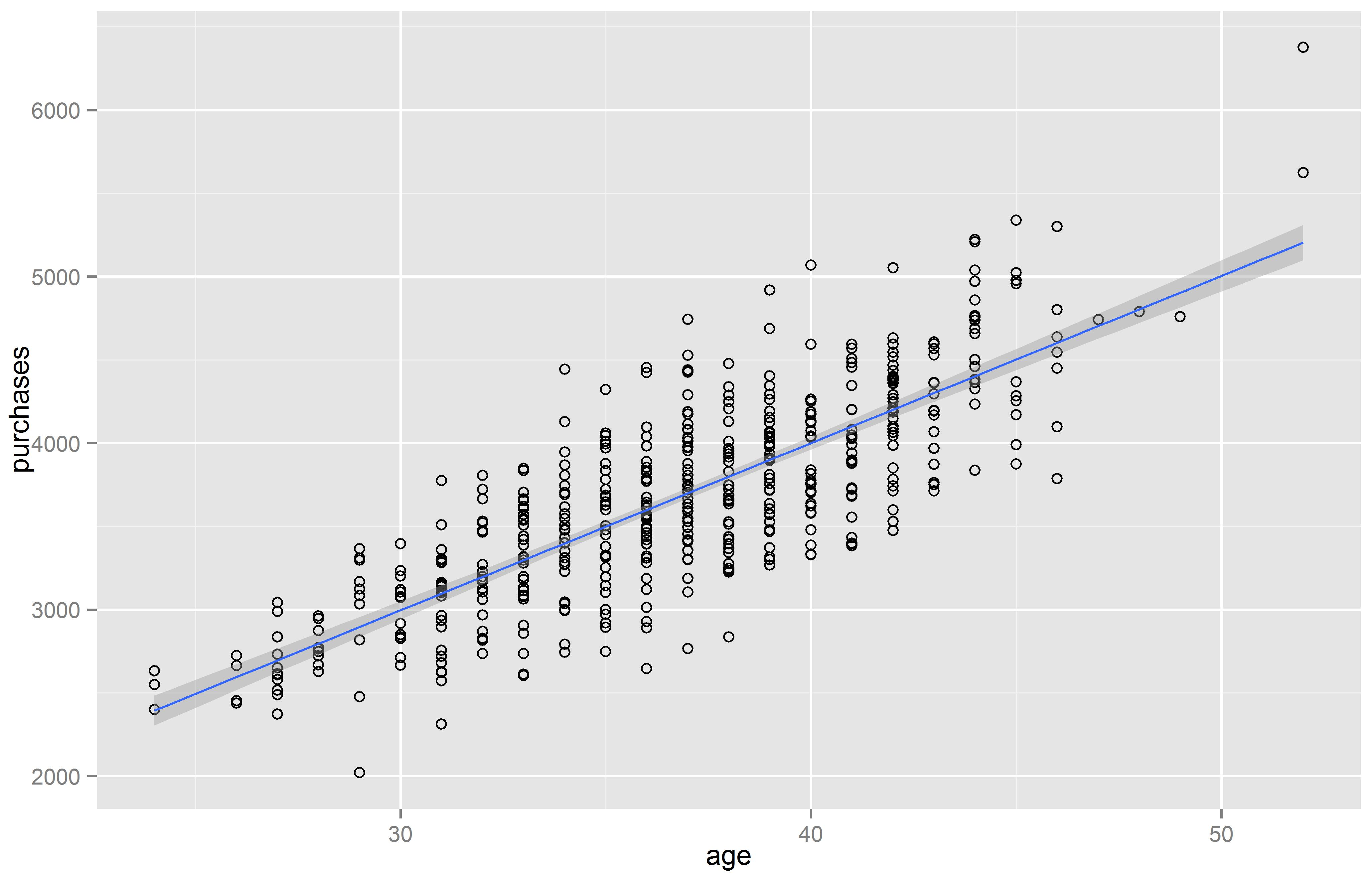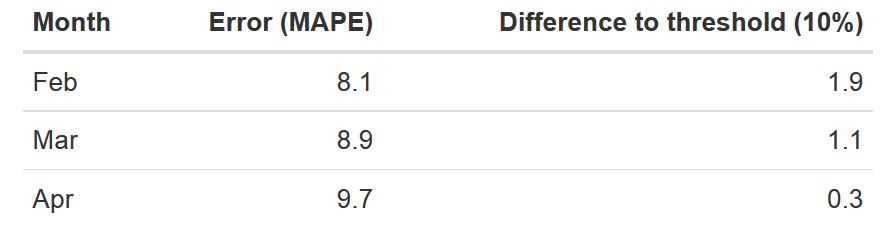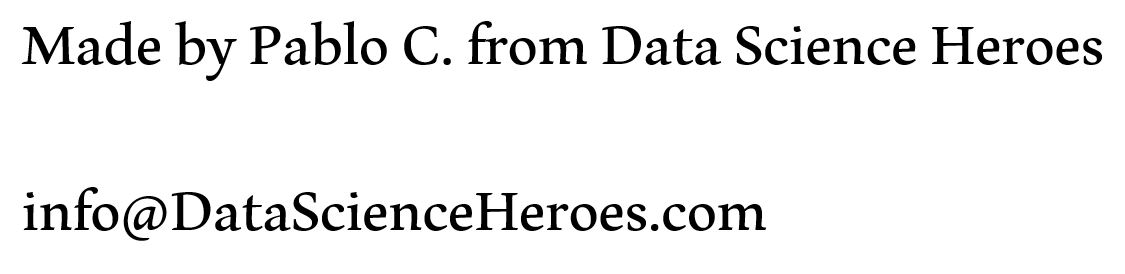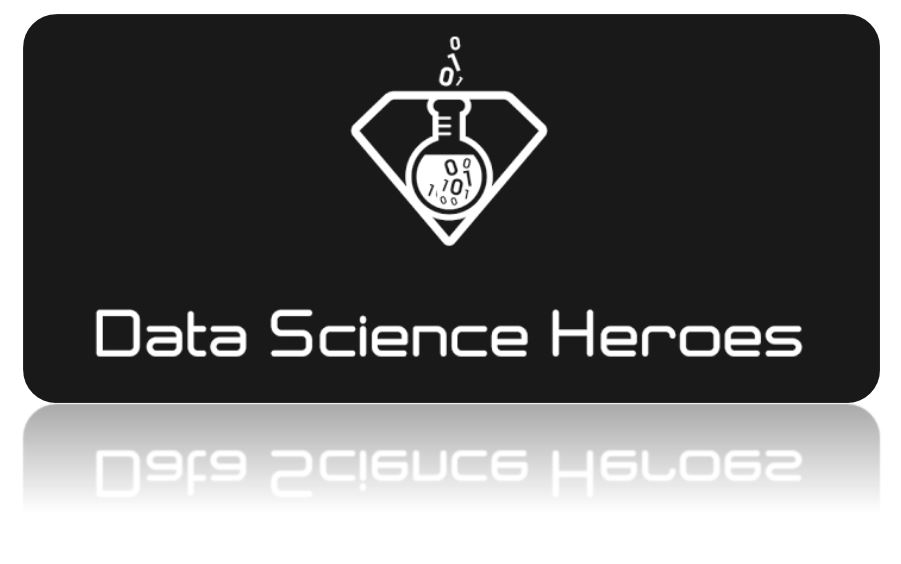[This article was first published on R - Data Science Heroes Blog, and kindly contributed to R-bloggers]. (You can report issue about the content on this page here)
Want to share your content on R-bloggers? click here if you have a blog, or here if you don't.

# Automatic Machine Learning

### Introduction

I want to develop a model that automatically learns over time“, a really challenging objective. We’ll develop in this post a procedure that loads data, build a model, make predictions and, if something changes over time, it will create a new model, all with R.Picture credit: S.H Horikawa

This post intends to recreate as simple as possible the machine learning scenario: automatically creation of a predictive model with temporal concerns. It’s going to be kind of manual because the objective is to cover a little of the logic behind a machine that learns.

### Our case

We have one input (age) and one output variable (purchases). We want to predict next months purchases based on age. If the model is inaccurate, a new one should be built.

#### Temporality

In machine learning, it’s quite important to understand temporality. We will stand in 3 different dates to introduce this concept:

• 1 Model building (January)
• 2 Model perfoming ok (February to April)
• 3.1 Model perfoming bad (May)
• 3.2 New model building (May)

### Step 1: Model building (January)

We’re on January, and we’re building the model with historical data, when we know both variables, age and purchases.

```## Loading needed libraries
suppressMessages(library(ggplot2))
suppressMessages(library(forecast))
```

Find the data sets used in this example in Github

```## Reading historical data
set.seed(999)

## Plotting current relationship between age and purchases

ggplot(data_historical, aes(x=age, y=purchases)) +
geom_point(shape=1) + ## Points as circles (good to see density)
geom_smooth(method=lm) ## Linear regression line

## Model creation. Input variable: "age", to predict "purchases".

model=lm(purchases~age, data=data_historical)
```Probably you know the linear regression, but if you don’t, check this.

Clearly the relationship between age and purchases is linear. After building the linear regression model, we check one accuracy metric: MAPE (Mean Average Percentage Error), close to 0, better.

MAPE measures how different is the prediction against the real value (in terms of percentage).

```## Checking accuracy model
historical_error=round(accuracy(model)[,"MAPE"],2)
historical_error

## Setting up error threshold (to be used later)
threshold=10 ## 10 represents "10%" of error (MAPE)
```
• MAPE in historical data is: 7.97% (historical_error variable).

It is expected to have a similar value over next months, if not, the model is not a good representation of reality.

Defining threshold:

There is the need to define an error threshold value, let’s say if the error (measuring by MAPE) in the following months is higher than 10%, model has to be rebuilding.

This rebuilding is the key point here, we can automate the process to take new data, build a new model, and if this new model has an error below threshold, then it becomes the new model in production, (the simplest scenario)

```## Checking model coefficients
model\$coefficients
```

R output:

```(intercept)    age
-15.4992    100.3812```

In other words, this is how the model looks like:
purchases=100.3812*age – 15.4992

### Step 2: Model performing ok (February to April)

During this period new customers arrive, the model to forecast purchases is applied the first day of each month. As a matter of fact we know how the model performed during this 3-month period, looking at real error (MAPE): predicted purchases vs. real purchases.

Note: Performance simulation and re-building with R code will be in next step (May)

Error table shows the following:As it can be seen, there’s an increasing tendency in error, getting closer to the maximum allowed.

### Step 3.1: Model performing bad (May)

Now we’re in May 31th. It is known how purchases were over current month. Following procedure should be executed at the end of every month.

```## Read data from past month, May.

## Retrieve the predictions made on May 1st based on the model built on January.
forecasted_purchases=predict.lm(object = model, newdata = data.frame(age=data_may\$age))

## Checking error
error_may=accuracy(forecasted_purchases, data_may\$purchases)[,"MAPE"]
error_may

## Difference to threshold (10%)
threshold-error_may
```

R output says:

“error_may” is 18.79473, and “threshold-error_may” is -8.794733In this month the error exceed the threshold by 8.79%.

This is how the model is working on May:

```## Further inspection plotting forecasted (blue) against actual (black) purchases.

ggplot(data_may, aes(x=age)) +
geom_line(aes(y=forecasted_purchases), colour="blue") +
geom_point(aes(y=purchases), shape=1)
```### Step 3.2: Model rebuilding

Clearly, the model works well predicting purchases on customers before 35 years-old, and becomes missaccuarate for older people. This segment is buying more than before.

It could be caused for example because of some change on business policy, a discount which is no more available, etc.

A new model must be created returning new error metrics.

```## Procedure to generate a new model
if(error_may>threshold) {

## Build new model, based on new data.
new_model=lm(purchases~age, data=data_may)

## Assign predictions to 'May' data. They are the predictions for training data.
data_may\$forecasted_purchases=new_model\$fitted.values

## Plot: new Linear regression
p=ggplot(data_may, aes(x=age)) +
geom_line(aes(y=forecasted_purchases), colour="blue") +
geom_point(aes(y=purchases), shape=1)

print(p)

new_error=accuracy(new_model)[,"MAPE"]

if(new_errorR output:
"We have a new model built in an automated process! =)"
We have the new model to run next month (June):
## Checking new model coefficients
new_model\$coefficients
R output:
(intercept)    age
-4414.1504    244.8179
In other words...
purchases=244.8179*age - 4414.1504

And there is the final model!

When a variable changes its distribution, affecting significantly  prediction accuracy, the model should be checked (in our case, 10%).

Other case is when a new variable appears, one that we didn't know when the model was built. Most advanced systems take care of this and automatically map this new concept. Like a search engine with new terms.

The most important point here is the concept of closed-system: The error is checked every month and determines if the model has or not to be re-adjusted.

One step ahead is to use the error to iteratively adapt the model (for example, testing other type of models, with other parameters) until the minimum error is reached.
Something similar to Artificial Neural Networks model, which measures error iteratively (hundreds or thousands of times...) to have a proper balance between generalization and particularization.

Finally...

If you want to improve your analytical skills, continue with the e-learning & self-paced course  Data Science with R which covers from data munging to predictive modelling -from basis. 1-to-1 Instructor Support, free demo available.

Linkedin group, post questions and/or share something related to data science. Share if you like 😉var vglnk = {key: '949efb41171ac6ec1bf7f206d57e90b8'};
(function(d, t) {
var s = d.createElement(t);
s.type = 'text/javascript';
s.async = true;
// s.defer = true;
var r = d.getElementsByTagName(t);
r.parentNode.insertBefore(s, r);
}(document, 'script'));

Related
ShareTweet